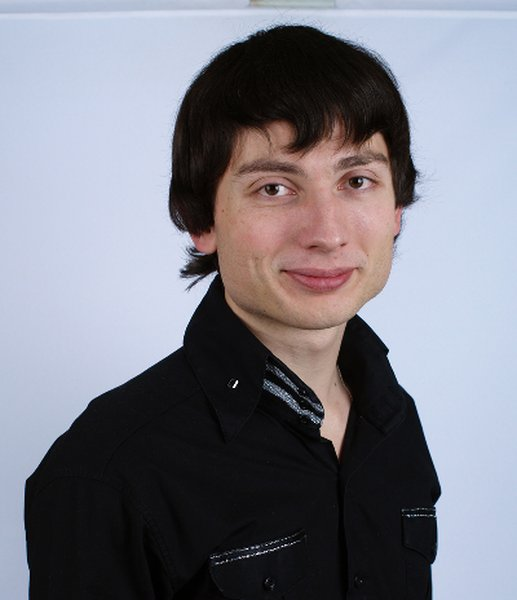Andriy V. Anikushin
Assistant Professor

### Information

 Position: Assistant Professor Candidate of Physical and Mathematical Science 215 259-05-32 linear operator equations, differential and integrodifferential partial differential equations, functional analysis

### Courses:

Mathematical analysis
Functional analysis
Complex analysis
Systems with distributed parameters

### Publications:

1. A. Anikushyn, M. Pokojovy Multidimensional Thermoelasticity for Nonsimple Materials – Well-Posedness and Long-Time Behavior, Applicable Analysis, Vol. 96 (2017), pp. 1561–1585

2. A. Anikushyn, D. Nomirovskyi, Qualitative analysis of linear differential pseudohyperbolic equations in some classes of distributions , Bulletin of Taras Shevchenko National University of Kyiv. Series: Phys. and Math. Sciences, No. 2 (2015), pp. 31–36 (in Ukrainian)

3. A. Anikushyn, On generalized solvability of the one integro-differential equation, J. Num. and App. Math., No. 2 (2014), pp. 25–32 (in Ukrainian)

4. A. Anikushyn, A. Hulianytskyi Generalized solvability of parabolic integro-differential equations, Differential Equations, Vol. 50 (2014), pp. 98–109

5. A. Anikushyn, Generalized optimal control for systems described by linear integro-differential equations with nonnegative definite integral operators, Journal of Automation and Information Sciences, Vol. 46 (2014), pp. 58–67

6. A. Anikushyn, Generalized solvability of linear hyperbolic integro-differential equation, Bulletin of Taras Shevchenko National University of Kyiv. Series: Phys. and Math. Sciences, No. 4 (2013), pp. 60–65 (in Ukrainian)

7. A. Anikushyn, A barrelledness of pre-Hilbert spaces, J. Num. and App. Math., No. 4(2012), pp. 51–55 (in Ukrainian)

8. A. Anikushyn, D. Nomirovskyi, Generalized solutions for linear operators with weakened a priori inequalities, Ukrainian Mathematical Journal, Vol. 62 (2011), pp. 1175–1186

9. A. Anikushyn, On relations between the a priori inequalities and the weak a priori inequalities, J. Num. and App. Math., No. 4 (2010), pp. 3–8 (in Ukrainian)

10. A. Anikushyn, Optimal control for parabolic-like integro-differential system, J. Num. and App. Math., No. 3(2010), pp. 3–16 (in Ukrainian)